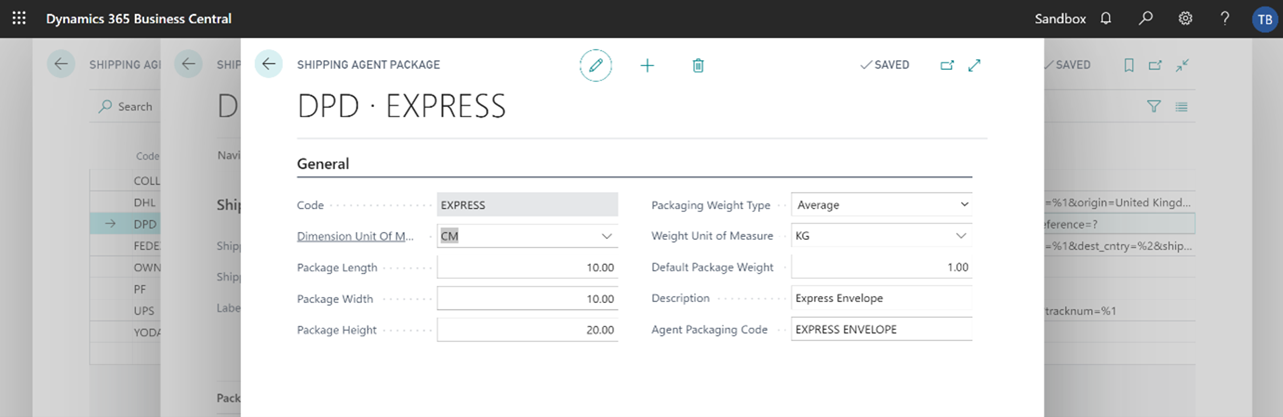For at least the default packaging option you must select the packaging option you’ve chosen from the Packaging section, then select Manage, Packaging Setup from the section toolbar.

You will see a page similar to the screenshot below and you need to complete the following fields

Dimension Unit of Measure: This needs the unit of measure that the Shipping Agent requires their dimensions in. Usually CM.

Package Length: The length of the package in the Dimension Unit of Measure selected.

Package Width: The width of the package in the Dimension Unit of Measure selected.

Package Height: The height of the package in the Dimension Unit of Measure selected.

Packaging Weight Type: Options of Default or Average. Where default is selected each package of this type that is consigned will default to the weight entered against the Default Package Weight below. Where Average is selected the system will add up the gross weight of all the items being consigned and add the Default Package Weight multiplied by the number of packages on the consignment to that. It then divides that total by the number of packages to give an average against each one.

Average Example

Item 1 weighs 0.5Kg each – we are sending 10

Item 2 weighs 0.25Kg each - we are sending 30

Total weight of all the items on the consignment is (0.5 x 10) + (0.25 * 30) = 12.5Kg

Default Package Weight is set to 0.2Kg and we are sending it two parcels (0.2 x 2) = 0.4

Total consigned Weight is 12.5 + 0.4 = 12.9Kg

We have 2 packages so the average is (12.9 / 2) = 6.45Kg for each parcel consigned.

Weight Unit of Measure: The Unit of Measure for Weight that needs to be used for this shipping Agent. Usually Kg.

Default Package Weight: If the Package Weight Type is Default then this is the default weight of a package often specified a slight amount below the maximum weight for this packaging type. E.g. 9.9Kg. If the Package Weight Type is Average then this should be set as the weight to add for packaging only to the item gross weight total before its divided by the number of packages.

Description: The usual description you use for this type of packaging i.e. Standard Box

Agent Packaging Code: Predefined as the packaging Code to register via the API.